Courses

# RD Sharma Solutions -Ex-18.2 (Part - 2), Surface Area And Volume Of A Cuboid And Cube, Class 9, Math Class 9 Notes | EduRev

## Class 9 : RD Sharma Solutions -Ex-18.2 (Part - 2), Surface Area And Volume Of A Cuboid And Cube, Class 9, Math Class 9 Notes | EduRev

The document RD Sharma Solutions -Ex-18.2 (Part - 2), Surface Area And Volume Of A Cuboid And Cube, Class 9, Math Class 9 Notes | EduRev is a part of the Class 9 Course RD Sharma Solutions for Class 9 Mathematics.
All you need of Class 9 at this link: Class 9

Q16) A box with lid is made of 2cm thick wood. Its external length, breadth and height are 25cm, 18cm and 15cm respectively. How much cubic cm of a fluid can be placed in it? Also, find the volume of the wood used in it.

Solution:

Given,

The external dimensions of cuboid are as follows

Length (l) = 25 cm

Heigth (h) = 15 cm

External volume of the case with cover (cuboid) = l*b*h cm3

= 25*18*15 cm3

= 6750 cm3

Now, the internal dimensions of the cuboid is as follows

Length (l) = 25-(2*2) = 21 cm

Breadth (b) = 18-(2*2) = 14 cm

Height (h) = 15-(2*2) = 11cm

Now, Internal volume of the case with cover (cuboid) = l*b*h cm3

= 21*14*11 cm3

= 3234 cm3

Therefore, Volume of the fluid that can be placed = 3234 cm3

Now, volume of the wood utilized = External volume – Internal volume

= 3516 cm3

Q17) The external dimensions of a closed wooden box are 48cm, 36cm, 30cm. The box is made of 1.5cm thick wood. How many bricks of size 6cm x 3cm x 0.75cm can be put in this box?

Solution:

Given that,

The external dimensions of the wooden box are as follows:

Length (l) = 48cm, Breadth (b) = 36cm, Heigth (h) = 30cm

Now, the internal dimensions of the wooden box are as follows:

Length (l) = 48-(2*1.5) = 45cm

Breadth (b) = 36-(2*1.5) = 33cm

Height (h) = 30-(2*1.5) = 27cm

Internal volume of the wooden box = l*b*h cm3

= 45*33*27 cm3

= 40095 cm3

Volume of the brick = 6*3*0.75 = 13.5 cm3

Therefore,Number of bricks =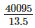= 2970 bricks

Therefore,2970 bricks can be kept inside the wooden box.

Q18) How many cubic centimeters of iron are there in an open box whose external dimensions are 36cm, 25cm and 16.5cm, the iron being 1.5cm thick throughout? If 1 cubic cm of iron weighs 15gms. Find the weight of the empty box in kg.

Solution:

Given,

Outer dimensions of iron:

Length (l) = 36cm

Heigth (h) = 16.5cm

Inner dimensions of iron:

Length (l) = 36-(2*1.5) = 33cm

Breadth (b) = 25-(2*1.5) = 22cm

Height (h) = 16.5-1.5 = 15cm

Volume of Iron = Outer volume – Inner volume

= (36*25*16.5) – (33*22*15)

= 3960 cm3

Weight of Iron = 3960*15 = 59400 grams = 59.4 kgs

Q19) A cube of 9cm edge is immersed completely in a rectangular vessel containing water. If the dimensions of the base are 15cm and 12cm, find the rise in water level in the vessel.

Solution:

Volume of the cube =  S3 = 93 = 729cm3

Area of the base = l*b = 15*12 =  180cm2

Risein water level =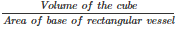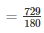= 4.05cm

Q20) A rectangular container, whose base is a square of side 5cm, stands on a horizontal table, and holds water up to 1cm from the top. When a solid cube is placed in the water it is completely submerged, the water rises to the top and 2 cubic cm of water overflows. Calculate the volume of the cube and also the length of its edge.

Solution:

Let the length of each edge of the cube be ‘x’cm

Then, volume of the cube = Volume of water inside the tank + Volume of water that overflowed

x= (5∗5∗1)+2

x3 = 27

x = 3cm

Hence, volume of the cube =  27cm3

And edge of the cube = 3cm

Q21) A field is 200 m long and 150 m broad. There is a plot, 50 m long and 40 m broad, near the field. The plot is dug 7m deep and the earth taken out is spread evenly on the field. By how many meters is the level of the field raised? Give the answer to the second place of decimal.

Solution:

Volume of the earth dug out = 50*40*7 = 14000 m3

Let ‘h’ be the rise in the height of the field

Therefore, volume of the field (cuboidal) = Volume of the earth dug out

⇒ 200∗150∗h = 14000

⇒ h =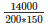= 0.47m

Q22) A field is in the form of a rectangular length 18m and width 15m. A pit 7.5m long, 6m broad and 0.8m deep, is dug in a corner of the field and the earth taken out is spread over the remaining area of the field. Find out the extent to which the level of the field has been raised.

Solution:

Let ‘h’metres be the rise in the level of field

Volume of earth taken out from the pit = 7.5*6*0.8 = 36 m3

Area of the field on which the earth taken out is to be spread = 18*15 – 7.5*6 = 225m2

Now, Area of the field * h = Volume of the earth taken out from the pit

⇒ 225∗h = 7.5∗6∗0.8

⇒ h =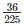= 0.16m = 16cm

Q23) A rectangular tank is 80m long and 25m broad. Water flows into it through a pipe whose cross-section is 25cm2, at the rate of 16 km per hour. How much the level of the water rises in the tank in 45 minutes?

Solution:

Consider ‘h’ be the rise in water level.

Volume of water in rectangular tank = 8000*2500*h cm2

Cross-sectional area of the pipe = 25 cm2

Water coming out of the pipe forms a cuboid of base area 25 cm2 and length equal to the distance travelled in 45 minutes with the speed 16 km/hour

i.e., length =  Length = 16000∗100∗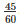cm

Therefore, The Volume of water coming out pipe in 45 minutes = 25*16000*100* (45/60)

Now, volume of water in the tank = Volume of water coming out of the pipe in 45 minutes

⇒ 8000∗2500∗h = 16000∗100∗4560∗25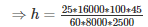= 1.5cm

Q24) Water in a rectangular reservoir having base 80 m by 60 m is 6.5m deep. In what time can the water be pumped by a pipe of which the cross-section is a square of side 20cm if the water runs through the pipe at the rate of 15km/hr.

Solution:

Flow of water = 15km/hr

= 15000 m/hr

Volume of water coming out of the pipe in one hour,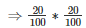∗15000 = 600m3

Volume of the tank = 80*60*6.5

= 31200 m3

Timetakentoemptythetank =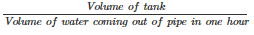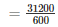= 52hours

Q25) A village having a population of 4000 requires 150 liters of water per head per day. It has a tank measuring 20m x 15m x 6m. For how many days will the water of this tank last?

Solution:

Given that,

Length of the cuboidal tank (l) = 20m

Breadth of the cuboidal tank (b) = 15m

Height of the cuboidal tank (h) = 6m

Capacity of the tank = l*b*h = 20*15*6

= 1800 m3

= 1800000 litres

Water consumed by the people of village in one day = 4000*150 litres

= 600000 litres

Let water of this tank last for ‘n’ days

Therefore, water consumed by all people of village in n days = capacity of the tank

= n*600000 = 1800000

=  n =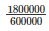= 3

Thus, the water will last for 3 days in the tank.

Q26) A child playing with building blocks, which are of the shape of the cubes, has built a structure as shown in Fig. 18.12. If the edge of each cube is 3cm, find the volume of the structure built by the child.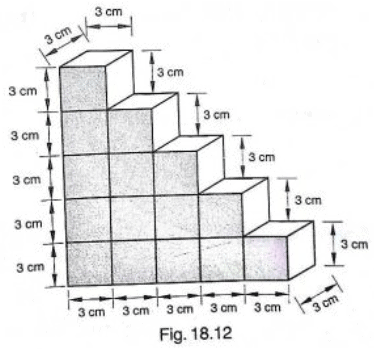Solution:

Volume of each cube = edge*edge*edge

= 3*3*3

= 27 cm3

Number of cubes in the structure = 15

Therefore, volume of the structure = 27*15

= 405 cm3

Q27) A godown measures 40m x 25m x 10m. Find the maximum number of wooden crates each measuring 1.5m x 1.25m x 0.5m that can be stored in the godown.

Solution:

Given,

Godown length (l1) = 40m

Godown height (h1) = 10m

Volume of the godown = l1* b1* h = 40*25*10

= 10000 m3

Wooden crate length (l2) = 1.5m

Wooden crate breadth (b2) = 1.25m

Wooden crate height (h2) = 0.5m

Volume of the wooden crate = l2*b2*h = 1.5*1.25*0.5

= 0.9375 m3

The number of wooden crates stored in the godown is taken as ‘n’

Volume of ‘n’ wooden crates = Volume of godown

= 0.9375n = 10000

=  n =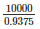= 10666.66

Therefore, the number of wooden crates that can be stored in the godown is 10666.66.

Q28) A wall of length 10m was to be built across an open ground. The height of the wall is 4m and thickness of the wall is 24cm. If this wall is to be built up with bricks whose dimensions are 24cm x 12cm x 8cm, how many bricks would be required?

Solution:

Given that,

The wall with all its bricks makes up space occupies by it, we need to find the volume of the wall, which is nothing but cuboid.

Here, length = 10m = 1000cm

Thickness = 24cm

Height = 4m = 400cm

Therefore, volume of the wall = l*b*h

= 1000*24*400 cm3

Now, each brick is a cuboid with length = 24cm

Height = 8cm

So, volume of each brick = l*b*h = 24*12*8 = 2304 cm3

The number of bricks required is given by,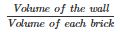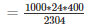= 4166.6 bricks

So, the wall requires 4167 bricks.

Offer running on EduRev: Apply code STAYHOME200 to get INR 200 off on our premium plan EduRev Infinity!

91 docs

,

,

,

,

,

,

,

,

,

,

,

,

,

,

,

,

,

,

,

,

,

,

,

,

,

,

,

,

,

,

;